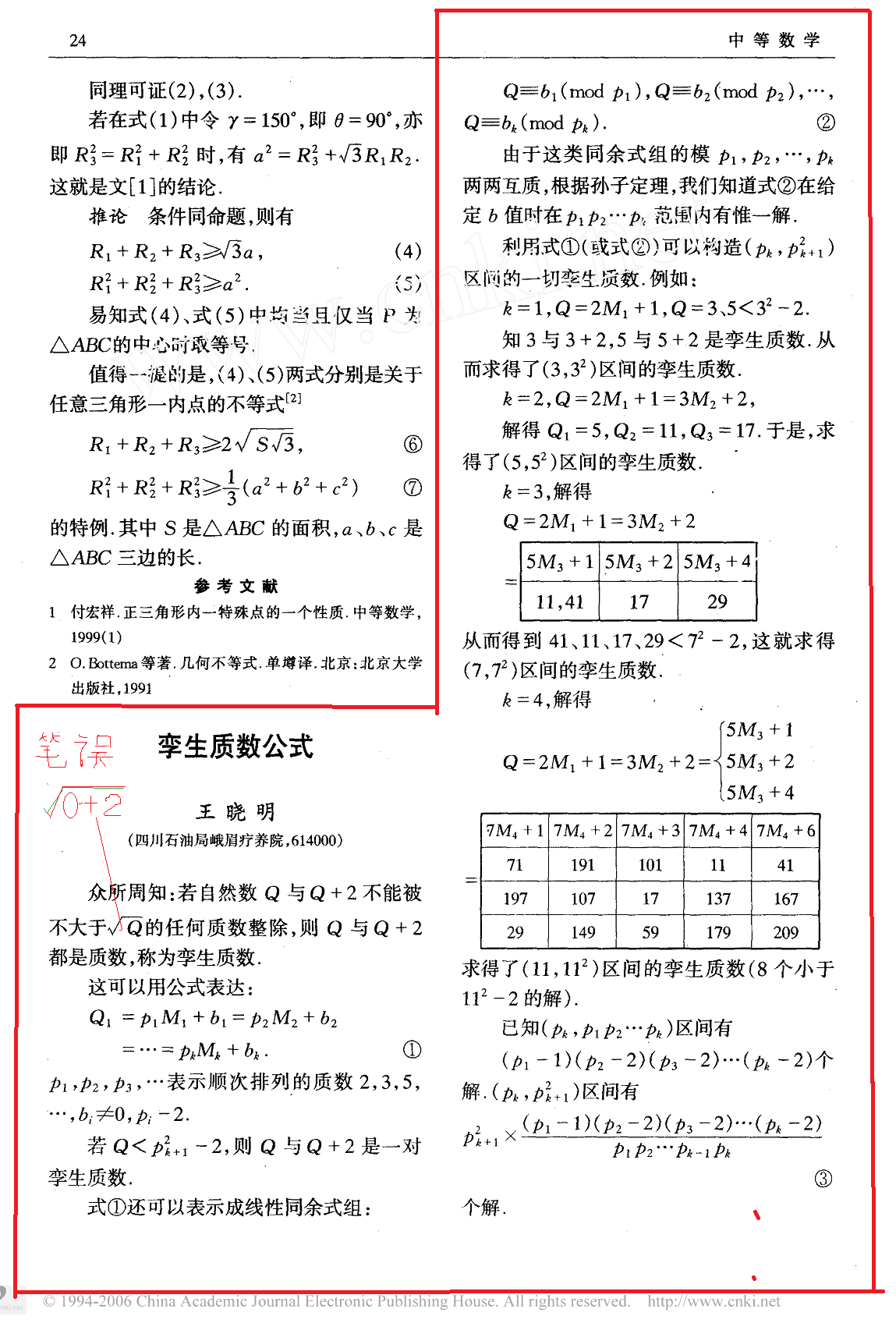• 汇集公众科学智慧交流科学思想见解
• 点燃科学智慧火花构建互动交流平台## 单卵双胞胎的数学模型——孪生素数公式

1、孪生素数的公式都是素数」。$q \equiv b_{1} \pmod{p_{1}}$，$q \equiv b_{2} \pmod{p_{2}}$，.... ，$q \equiv b_{k} \pmod{p_{k}}$....(2)

（2）式有唯一一个小于的正整数解。

2、范例，解得k=3時= 11和41 17 29）（）()...（）...（3）个解。注意，由于$b_{1}$≠0;$b_{1}$$p_{1}-2$是一回事所以第一项（$p_{1}-1$）。

3、结论推广